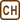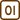## Water in Tropical Seas

### Task number: 1801

Water in tropical seas is much warmer at the surface than at depth. Is it possible to construct a heat engine that would work between the warm and cold water? If so, can you estimate its efficiency? If not, why not?

• #### Hint – efficiency η

A heat engine working between hot and cold body with fixed temperatures reaches maximum efficiency, provided that it works according to the ideal Carnot cycle. This efficiency is given by the relation

$\eta_{id}=\frac{T_1-T_2}{T_1},$

where T1 is the temperature of water at the surface (hot reservoir), and T2is the temperature at depth (cold reservoir).

• #### Hint – temperature of water

Try to estimate or find on the Internet the temperature of sea water at surface and at depth.

• #### Solution

Theoretically, such an engine would be possible to construct. Its effectiveness, however, would be very small.

Even in the case that this machine would work according to the ideal Carnot cycle (which in this case cannot possibly happen), its efficiency would be given by the relation

$\eta_{id}=\frac{T_1-T_2}{T_1},$

where T1 would be the temperature of the surface water, and T2 the temperature at depth.

We estimate the temperature of the sea at the surface to be 30 °C (303.15 K) and the temperature at depth to be 4 °C (277.15 K), we obtain a very small efficiency according to the formula above:

$\eta_{id}=\frac{T_1-T_2}{T_1}=\frac{303.15-277.15}{303.15}\dot{=}0.086,$

or approximately 8.6 %.

In reality, this value is much smaller. Moreover, it would be extremely difficult to construct such an engine. Therefore, the attempt to construct a heat engine like this would be futile.